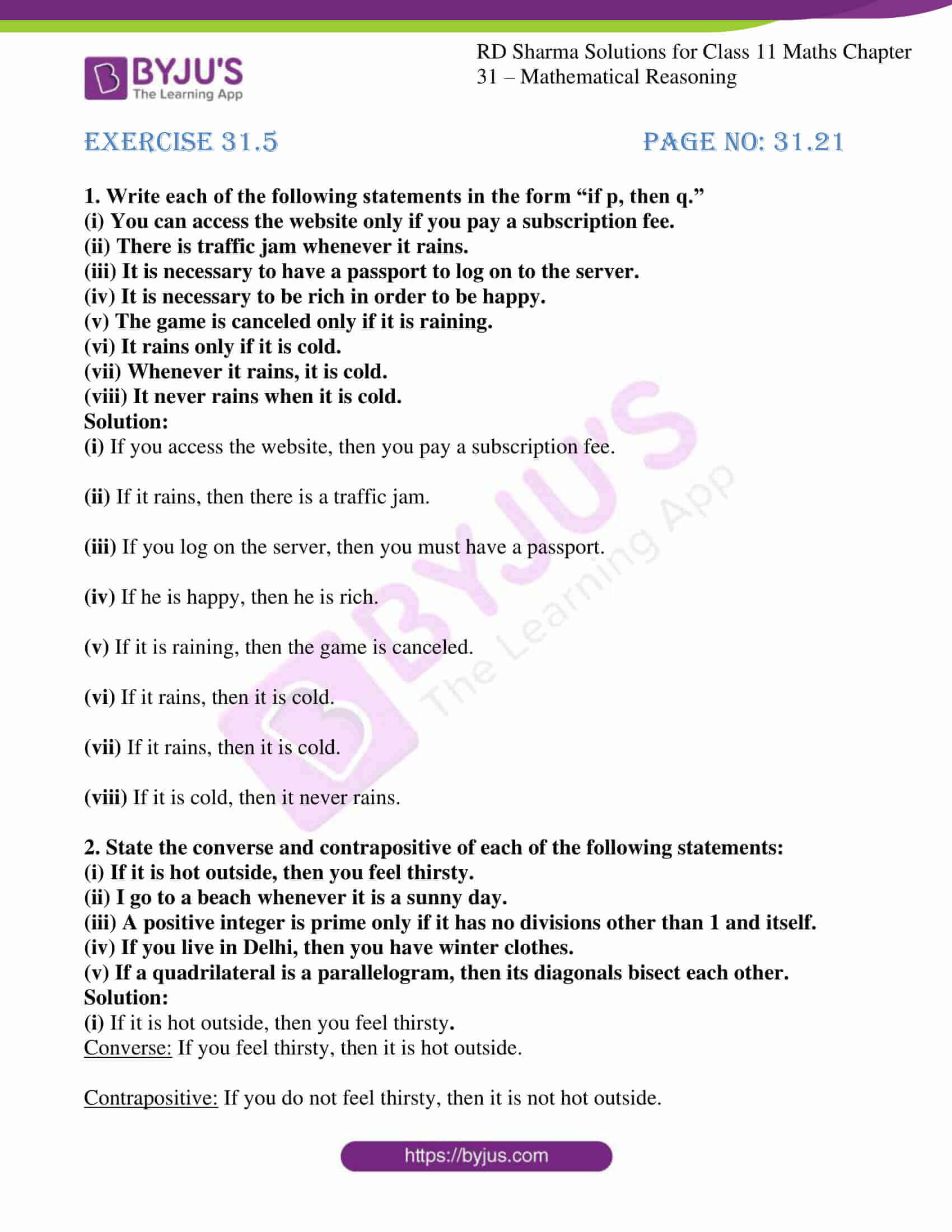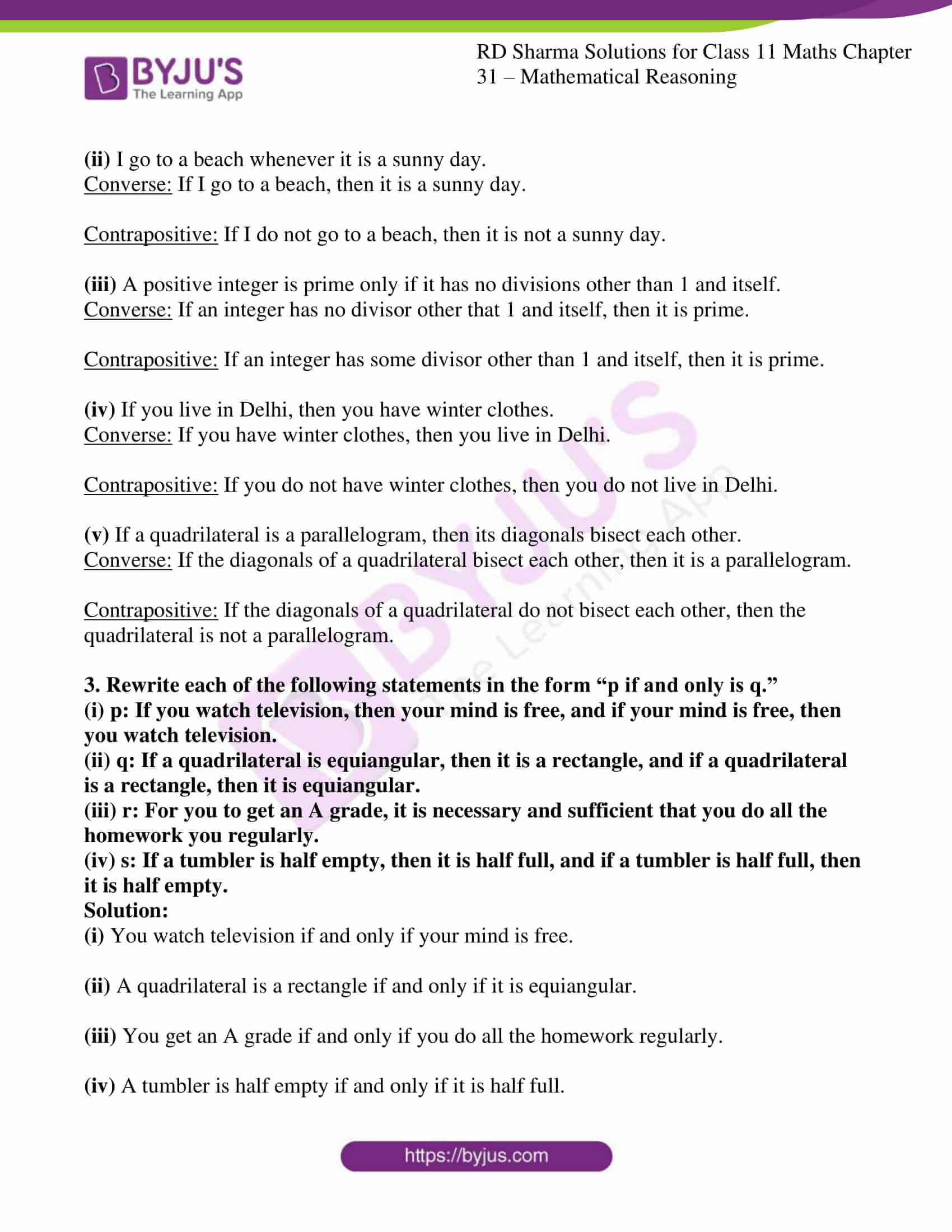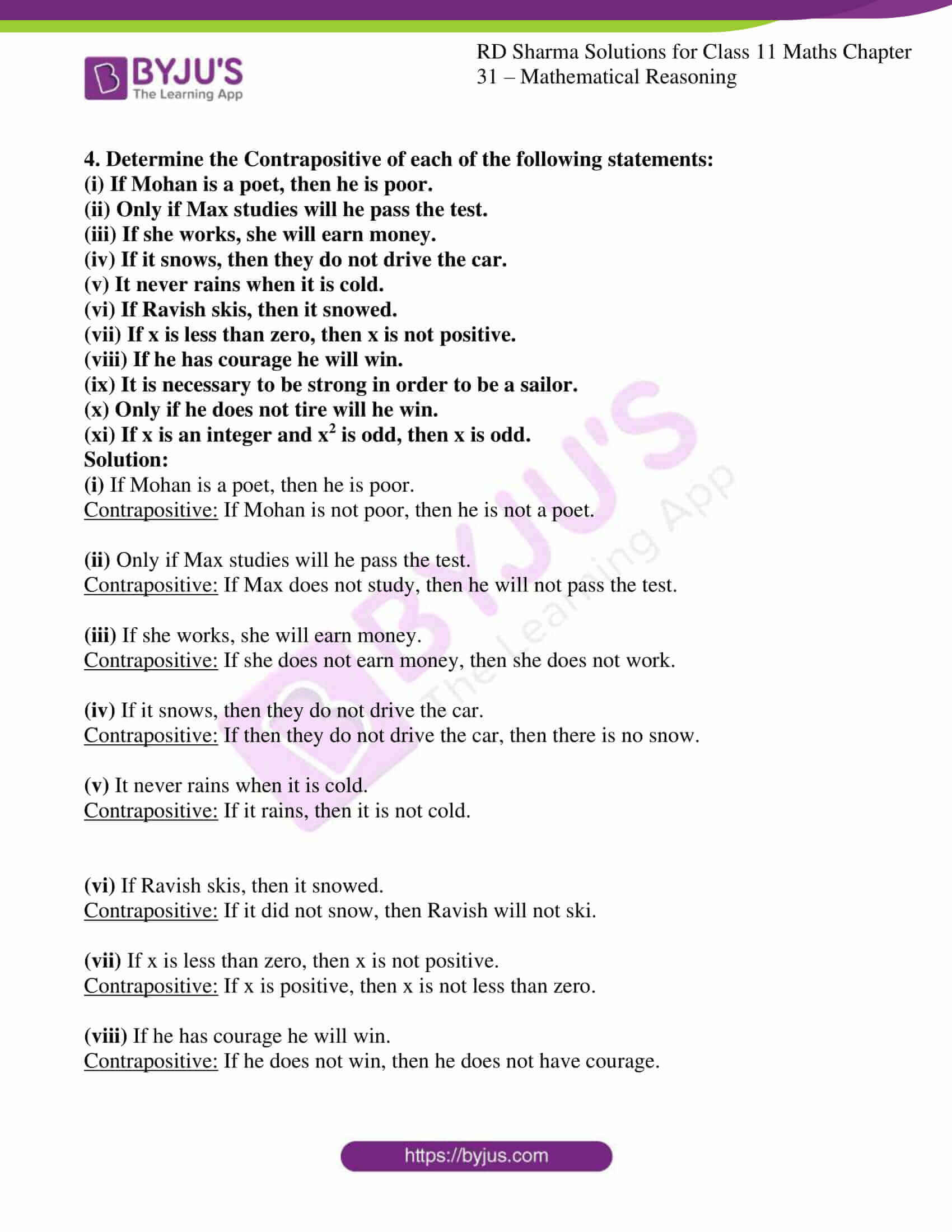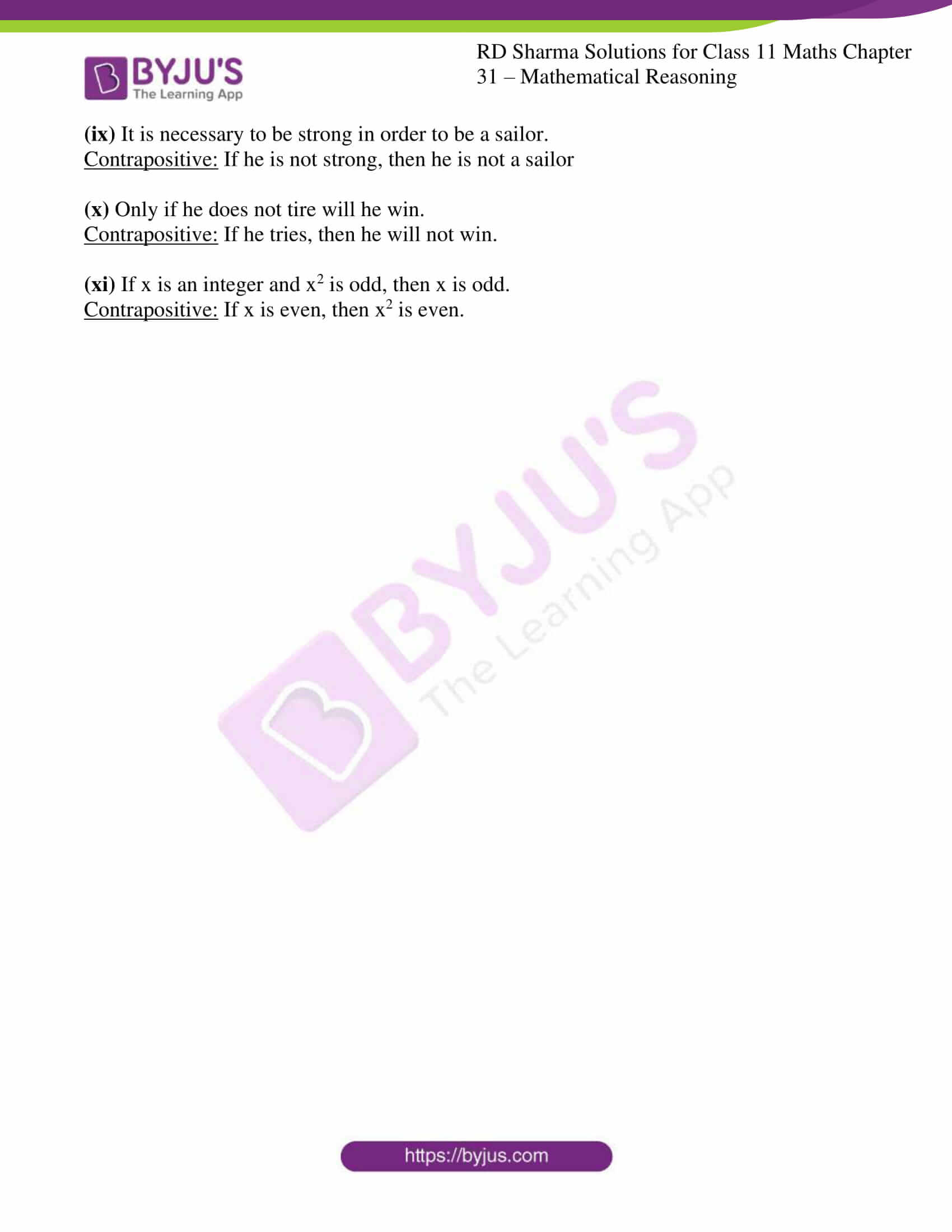# RD Sharma Solutions for Class 11 Chapter 31 - Mathematical Reasoning Exercise 31.5

In mathematics we come across many statements of the form “if p then q”, “p only if q”, and “if and only if” such statements are called implications. In this section, we shall discuss such statements. The solutions are prepared and reviewed by the subject matter experts and it is revised according to the latest syllabus. For students to excel in the board examinations, these solutions will increase their confidence level, as the concepts are clearly explained and structured. RD Sharma Class 11 Maths Solutions are widely used by students who wish to excel in board exams as it provides a vast number of questions to solve. Students can refer and download the pdf of RD Sharma Solutions which is available in the links given below.

## Download the pdf of RD Sharma Solutions for Class 11 Maths Exercise 31.5 Chapter 31 – Mathematical Reasoning